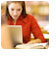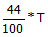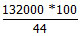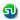### CAT, XAT, FMS, IIFT, SNAP entrance Math sample questions - Percentages - Ascent Education MBA Entrance preparation

 Home TANCET Classes GMAT Coaching Online GMAT Course CAT Classes TANCET Study Material
 TANCET '18 Classroom ProgramWeekend and weekday classes for TANCET MBA @ Chennai. At Nungambakkam and Velachery.Other Courses TANCET Correspondence Course CAT Classes & Courses GMAT Classes Chennai Online GMAT Course GRE Classes Chennai CBSE Math Online Tuition SAT Classes Other Links Ascent TANCET Toppers Testimonials CAT, TANCET Questions Careers @ Ascent Contact Us +91 44 4500 8484 +91 96000 48484 ascent@ascenteducation.com Postal Address Facebook / Twitter / Blog / Videos
You are here: Home  »  XAT, TANCET Prep Questions »  Percents, Fractions »   Question 3

# Percentages - Quant/Math - XAT 2016 Practice

This question is from the topic Percentages.

## Question

In an election contested by two parties, Party D secured 12% of the total votes more than Party R. If party R got 132,000 votes and there are no invalid votes, by how many votes did it lose the election?
1. 300,000
2. 168,000
3. 36,000
4. 24,000

36,000. Choice (3)

Let the percentage of the total votes secured by Party D be x%
Then the percentage of total votes secured by Party R = (x - 12)%

As there are only two parties contesting in the election, the sum total of the votes secured by the two parties should total up to 100%

i.e., x + x - 12 = 100
2x - 12 = 100
or 2x = 112 or x = 56%.

If Party D got 56% of the votes, then Party got (56 - 12) = 44% of the total votes.

44% of the total votes = 132,000
i.e.,= 132,000
T == 300,000 votes.

The margin by which Party R lost the election = 12% of the total votes
= 12% of 300,000 = 36,000.

Correct Answer : Choice (3)

## CAT, XAT, TANCET Practice Questions and Answers : Listed Topicwise

 Number Theory Permutation Combination Probability Inequalities Geometry Mensuration Trigonometry Coordinate Geometry Percentages Profit Loss Ratio Proportion Mixtures Alligation Speed Time Distance Pipes Cisterns Interest Races Average, Mean AP, GP, HP Set Theory Clocks Calendars Algebra Function English Grammar General Awareness Data sufficiency TANCET Papers XAT PapersAdd to del.icio.usStumble Itdigg this